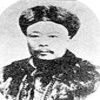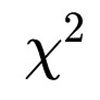#### You may also like### Very Old Man

Is the age of this very old man statistically believable?### Reaction Timer Timer

How can you time the reaction timer?### Chi-squared Faker

How would you massage the data in this Chi-squared test to both accept and reject the hypothesis?

# Powerful Hypothesis Testing

##### Age 16 to 18Challenge Level
Robin has a bag containing red and green balls.  Robin wants to test the following hypotheses, where $\pi$ is the proportion of green balls in the bag:

$H_0\colon \pi=\frac{1}{2}$  and  $H_1\colon \pi\ne\frac{1}{2}$

Robin is allowed to take out a ball at random, note its colour and then replace it: this is called a trial.  Robin can do as many trials as desired.

Robin uses the following approach:

"I will do exactly 50 trials.  If the p-value* is less than 0.05, then I will reject the null hypothesis at the 5% significance level, otherwise I will accept it."

If the null hypothesis is false, what is the probability that the null hypothesis will be rejected?

You can explore this question with the following simulation.
Warning - the computer needs a little bit of thinking time to do the simulations!

In this simulation, you can:
• specify the number of green and red balls actually in the bag - note that in a real experiment we would not know this!
• specify the number of trials per experiment (up to 200)
• specify the proportion for the null hypothesis (which we took to be $\frac{1}{2}$ above)
• repeat the experiment
Start by running the simulation a few times.
Now try changing the settings.  Can you predict what will happen as a result of your changes?

Here are some further questions you could consider:
• What is the probability of $H_0$ being rejected?
• If $H_0$ is rejected, how likely is it that the alternative hypothesis $H_1$ is true?
• the true proportion of greens in the bag changes?
• the significance level changes?
• the hypothesised proportion $\pi$ changes?

If Robin wants to be 90% certain of rejecting the null hypothesis if it is wrong, how many trials are needed?

You may want to ask and explore other questions as well.

The probability of correctly rejecting $H_0$ when it is false is called the power of the test.  Accepting $H_0$ when it is false is called a Type II error.

* If you want to read about what p-values are, have a look at What is a Hypothesis Test?. In this case, the p-value is calculated like this: after all of the trials, we find twice the probability of obtaining this number of greens or a more extreme number, assuming that $H_0$ is true.  For more on the effect of different ways of choosing the number of trials to perform, see Robin's Hypothesis Testing.

This resource is part of the collection Statistics - Maths of Real Life